# 14.7.2 BP and RP Spectra Validation

## standard_deviation and chi_squared

We investigate the dependence of bp_standard_deviation, rp_standard_deviation, bp_chi_squared, and rp_chi_squared from $G$ and $G_{\mathrm{BP}}-G_{\mathrm{RP}}$. For this test we use the 6377 stellar members of 17 open clusters whose main sequences are clearly visible in a CMD (i.e., IC 4651, Melotte 20, Melotte 22, NGC 2099, NGC 2168, NGC 2287, NGC 2506, NGC 2516, NGC 2632, NGC 3114, NGC 3532, NGC 3766, NGC 457, NGC 6405, NGC 6475, Stock 2, Trumpler 19). These members of open clusters have been identified by Cantat-Gaudin et al. (2020).

A single open cluster is a group of stars with similar bp_n_measurements and rp_n_measurements (see top panels in Figure 14.84) therefore variations in bp_standard_deviation, rp_standard_deviation, bp_chi_squared, and rp_chi_squared are mainly due to factors such as luminosity and colour.

As expected, both bp_standard_deviation and bp_standard_deviation/bp_n_measurements decrease as a function of $G$ (see mid and bottom panels in Figure 14.84). The same happens for the RP channel.

However, we also observe that these quantities can be higher than expected for stars with $1.0. This behaviour is evidenced in Figure 14.85, which shows bp_n_measurements, rp_n_measurements, bp_chi_squared, rp_chi_squared, bp_standard_deviation, and rp_standard_deviation as a function of $G_{\mathrm{BP}}-G_{\mathrm{RP}}$ for the 242 members of Melotte 20. Although there is no significant variation in n_measurements among the members of the cluster (top panels), Figure 14.85 shows that stars with $1.0 can have higher values of chi_squared (mid panels), and standard_deviation (bottom panels) than the typical values of the other cluster members. Besides Melotte 20, other clusters show a similar behaviour. However, that is not generalised to all clusters.

Interestingly, the enhanced standard_deviation and chi_squared values only characterise stars with $1.0 regardless of the clusters and its distance. Conversely, the luminosity regime of enhanced values is located at smaller $G$ for closer clusters and larger $G$ for the most distant ones. That indicates that only stars within the $1.0 regime can have enhanced quantities of standard_deviation and chi_squared. It also indicates that the behaviour does not depend on the stellar luminosity. The reason of that behaviour is still unknown.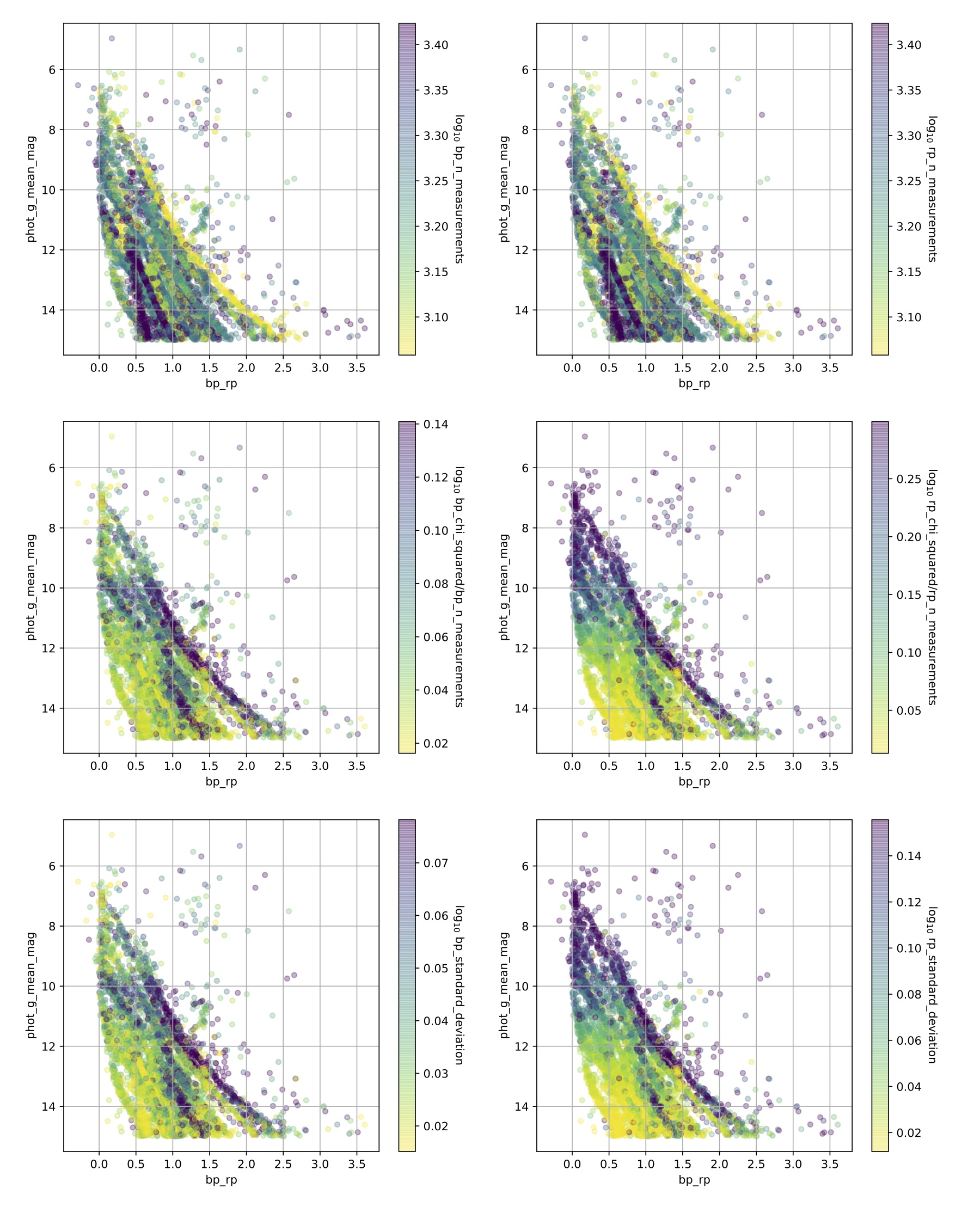Figure 14.84: CMD with stellar members of the 17 open clusters. Symbols are colour coded as a function of n_measurements, standard_deviation, and chi_squared. Left panels are relative to blue spectra, while right panels are relative to red spectra.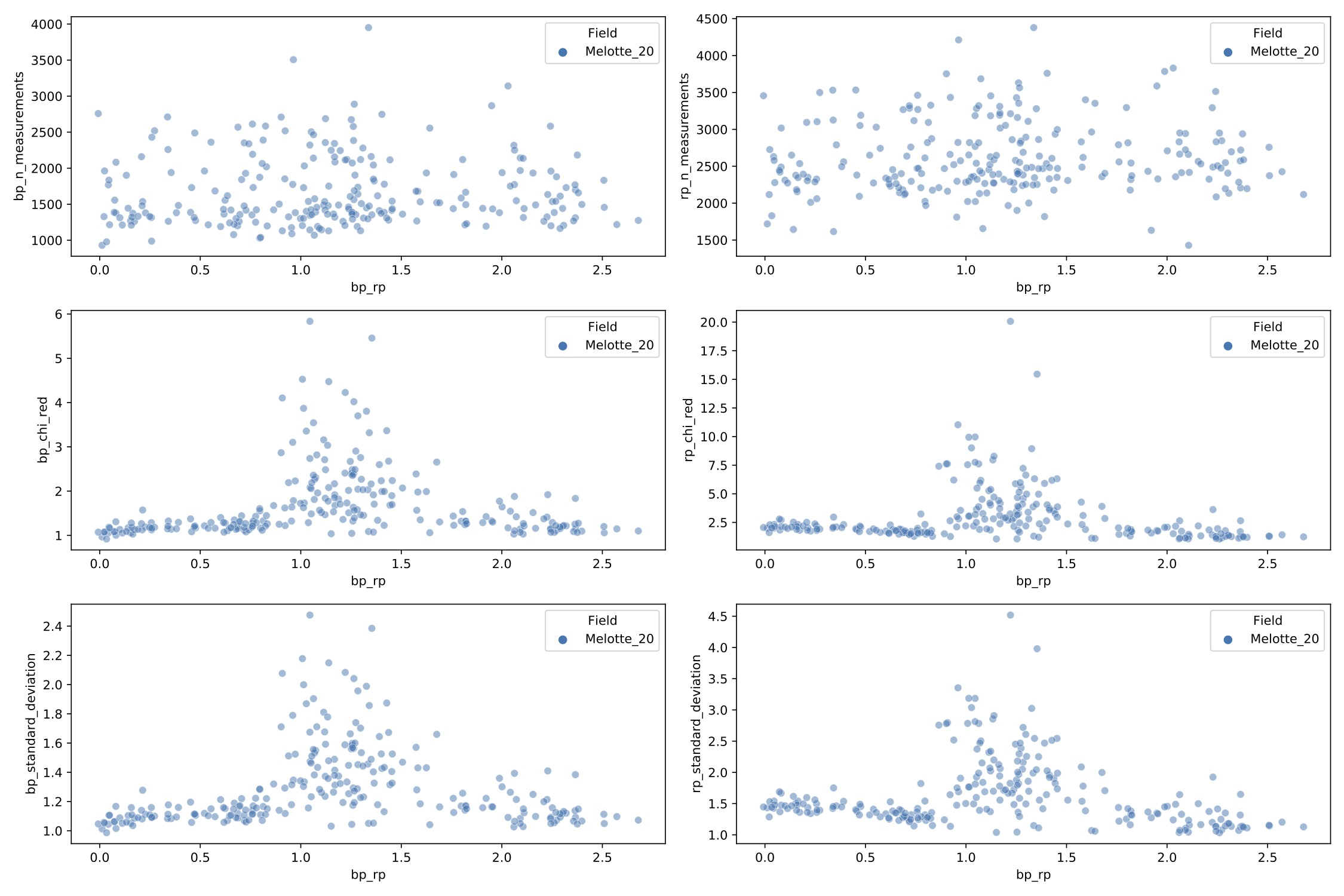Figure 14.85: n_measurements, standard_deviation, and chi_squared as a function of GBP-GRP for stellar members of Melotte 20. As in Figure 14.84, left panels are relative to blue spectra, while right panels are relative to red spectra.

## Wiggling and truncation

We tested if truncation efficiently removes unnecessary wiggling patterns across XP spectra. Here we considered 6377 main-sequence stellar members (Cantat-Gaudin et al. 2020) of 17 open clusters (i.e., IC 4651, Melotte 20, Melotte 22, NGC 2099, NGC 2168, NGC 2287, NGC 2506, NGC 2516, NGC 2632, NGC 3114, NGC 3532, NGC 3766, NGC 457, NGC 6405, NGC 6475, Stock 2, Trumpler 19). Since our testing sample is exclusively composed by stellar members of open clusters, we ensure that they all have similar metallicities around the Solar value. We employed XP spectra that were externally calibrated by GaiaXPy v0.0.5 with standard sampling.

First, we define a coefficient which measures the wiggling level in XP spectra. For each $i^{\rm th}$ wavelength sampled portion of the spectrum we calculate

 $\delta^{n}_{i}=\frac{|f_{i}-mean(f_{i-n},f_{i+n})|}{\texttt{phot\_g\_mean\_% flux}},$ (14.28)

where $f_{i}$ is the flux associated with the $i^{\rm th}$ wavelength sample. Therefore, the wiggling coefficient is defined as the average of the $\delta^{n}_{i}$ across the entire spectrum or a portion of it:

 $w_{n}=\sum_{i}\frac{\delta^{n}_{i}}{N},$ (14.29)

where $N$ is the number of wavelengths over which $\delta^{n}_{i}$ is determined.

The wiggling coefficient $w_{3}$ has been calculated for each star within the spectral range [450, 900] nm. This coefficient is higher when the spectra contains more undesired wiggles, but also for later spectral types whose spectra are typically richer of molecular bands. Thus, in order to ensure that we probe the wiggling and not real spectral features, we define a differential coefficient

 $\Delta w_{3}=\log_{10}(w_{3})-\log_{10}(\overline{w_{3}})$ (14.30)

where $w_{3}$ is the coefficient defined in Equation 14.29 and measured for the $j^{\rm th}$ star, while $\overline{w_{3}}$ is the coefficient measure on the average spectrum calculated over a sample of 100 dwarf stars with $G=G_{j}\pm 0.01$ mag and $G_{\mathrm{BP}}-G_{\mathrm{RP}}=[G_{\mathrm{BP}}-G_{\mathrm{RP}}]_{j}\pm 0.005$. In fact, by averaging spectra stars with very similar $G$ and $G_{\mathrm{BP}}-G_{\mathrm{RP}}$ obtain a single spectrum which contains the typical absorption features that can be found in spectra of stars similar to the $i^{\rm th}$ star and cleaned from wiggles. Therefore, $\Delta w_{3}$ is truly representative of the actual wiggling carried by the $j^{\rm th}$ spectrum, without the contribution from molecular bands or any other spectral feature.

Figure 14.86 shows the cumulative histograms of the differential wiggling coefficients $\Delta w_{3}$ derived for stars in different bins of $G$. The coefficients derived from non-truncated spectra are plotted in the left panel, while those from truncated spectra are in the right panel. While fainter stars tend to have larger $\Delta w_{3}$ in their non-truncated spectra due to a lower signal-to-noise, this dependence is significantly reduced by truncation.

Similarly, we plot in Figure 14.87 the cumulative histograms of the differential wiggling coefficients $\Delta w_{3}$ derived for stars in different bins $G_{\mathrm{BP}}-G_{\mathrm{RP}}$. By comparing the left and right panels we appreciate how wiggling is significantly alleviated in truncated spectra.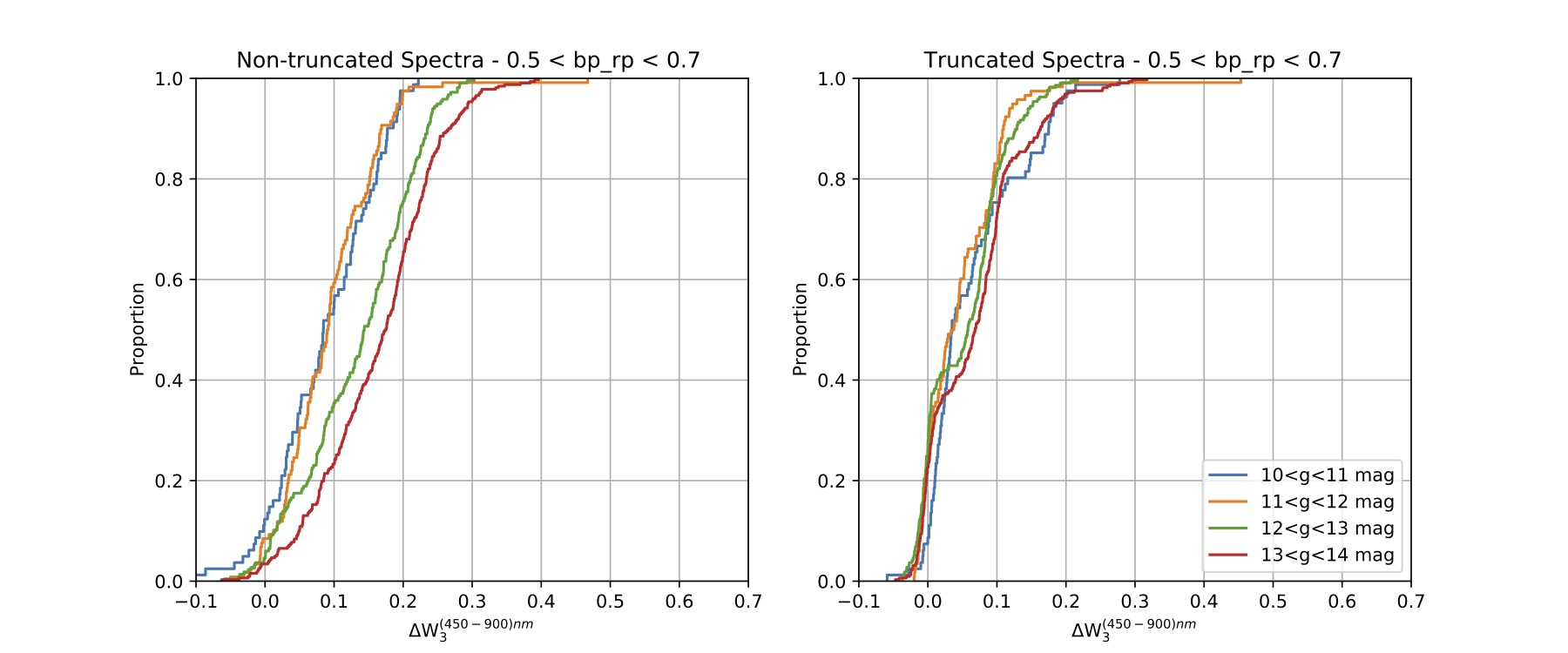Figure 14.86: Cumulative histogram of the differential wiggling coefficient Δ⁢w3 measured for stars with 0.5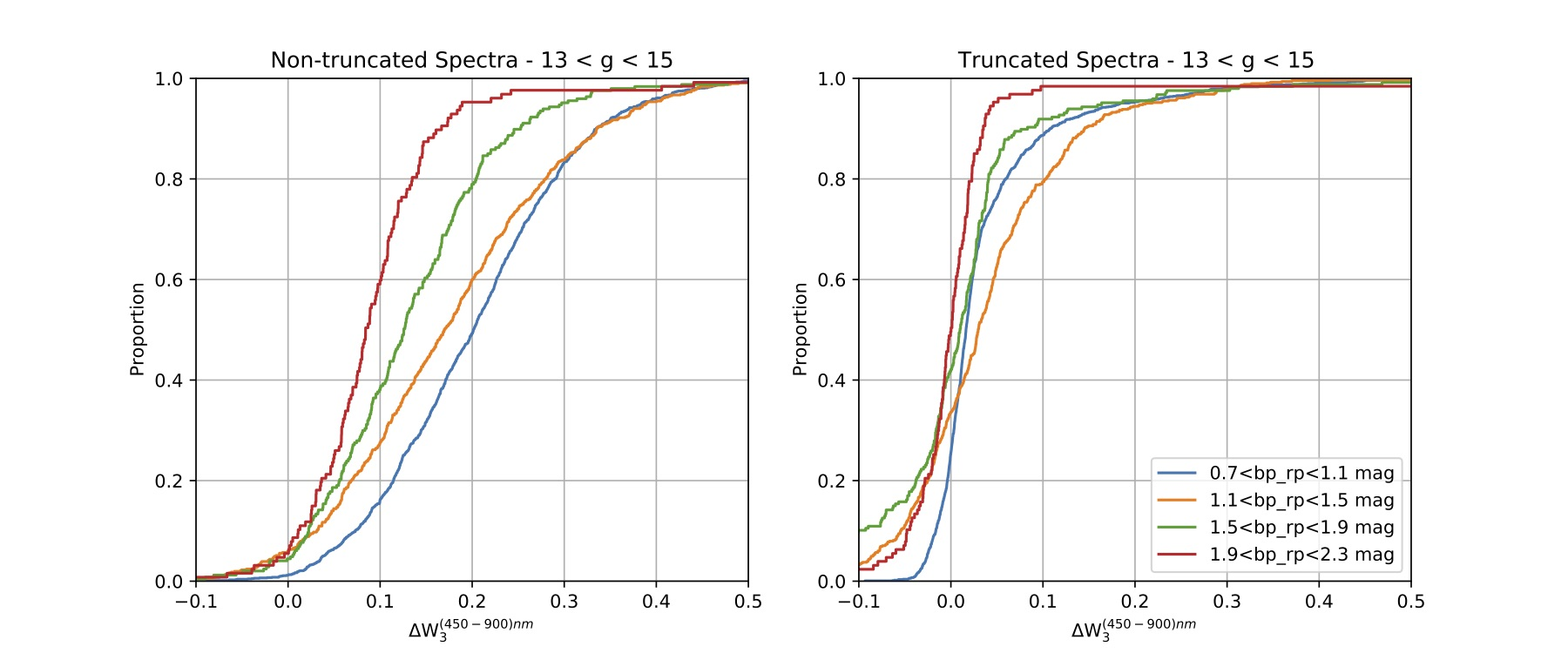Figure 14.87: Cumulative histogram of the wiggling factor Δ⁢w3 measured for stars with 13

## Wiggling and strong spectral features

Can wiggling in XP spectra be enhanced by strong spectral features? Testing this eventuality is especially important in spectra of young accretors which are typically characterised by strong H$\alpha$ emission lines. Therefore, for this test we used XP spectra from 197 members of the star forming regions Chamaeleon I, IC 348, Lupus, NGC 2024, NGC 2068, ONC, Ophiucus, and R Coronae Australis. The spectra were externally calibrated by GaiaXPy v0.0.5 with a standard sampling.

We define a coefficient which measures the height of the H$\alpha$ line:

 $H_{\mathrm{H}\alpha}=\frac{f_{\mathrm{H}\alpha}}{\langle(f_{(626-636)\cup(676-% 686)})\rangle},$ (14.31)

where f${}_{\mathrm{H}\alpha}$ is the flux measured at 656 nm, corresponding to the centre of the H$\alpha$ line, while the mean flux at the denominator is measured at the base of the H$\alpha$ line, i.e., at all wavelengths within 626-636 nm and within 676-686 nm.

The differential wiggling coefficient $\Delta w_{10}$ (see Equations 14.29 and 14.30) is shown in Figure 14.88-left as a function of H${}_{\mathrm{H}\alpha}$ for non-truncated spectra. Each error bar represents the standard deviation of the coefficient $\overline{w_{10}}$ measured on the comparison sample of 100 dwarfs. The plot shows that wiggling increases with the height of the H$\alpha$ line. Therefore, we conclude that the presence of strong spectral features enhance wiggling in XP spectra.

In order to we test whether truncation is able to fix or alleviate the problem, we repeated the experiment on truncated XP spectra. The results are shown in Figure 14.88-right panel, which indicates that truncation does not significantly remove the additional wigging produced by strong H$\alpha$ lines.

Instead, we found that the height of the H$\alpha$ line is affected by truncation. In Figure 14.89 we show the relative variation in H${}_{\mathrm{H}\alpha}$ height measured in the truncated and non-truncated spectra as a function of the H${}_{\mathrm{H}\alpha}$ from non-truncated spectra: we observe that the 23$\%$ of stars with H${}_{\mathrm{H}\alpha}^{non-trunc}>1.1$ have their H${}_{\mathrm{H}\alpha}$ reduced of more than 5$\%$ by truncation.Figure 14.88: Differential wiggling Δw10 as a function of HH⁢α. Non-truncated and truncated spectra as indicated.Figure 14.89: Relative variation of the HH⁢α between truncated and non-truncated spectra as a function of the HH⁢α measured on non-truncated spectra.

## Testing the uncertainties of the XP coefficients

This analysis performed on the coefficients of the BP/RP spectra aims at testing whether or not their uncertainties are correctly evaluated. To do so we compare those from pairs of stars using the following Equations:

 $\chi^{2}=(X\_1-X\_2)^{T}(C\_1+C\_2)^{-1}(X\_1-X\_2)$ (14.32)

where X_1 and X_2 are the coefficients of the two stars either in the BP or in the RP channels, while C_1 and C_2 are the associated covariance matrices. In order to ensure that we are comparing stars with same metallicity and reddening, we apply Eq. 14.32 only to pairs of stars belonging to the same open cluster (i.e., membership probability$\geq$0.7 from Cantat-Gaudin et al. 2020). We also excluded from the comparison all stars with ruwe $>$1.4 and those belonging to open clusters younger than 100 $\,\rm Myr$. This latter selection is necessary to avoid contamination due to differential extinction. Furthermore, the two stars must have $G$ and $G_{\mathrm{BP}}-G_{\mathrm{RP}}$ that are consistent within their uncertainties. Applying all these selection criteria we obtain a controlled sample of 1560 stellar pairs for which we can derive the $\chi^{2}$ values.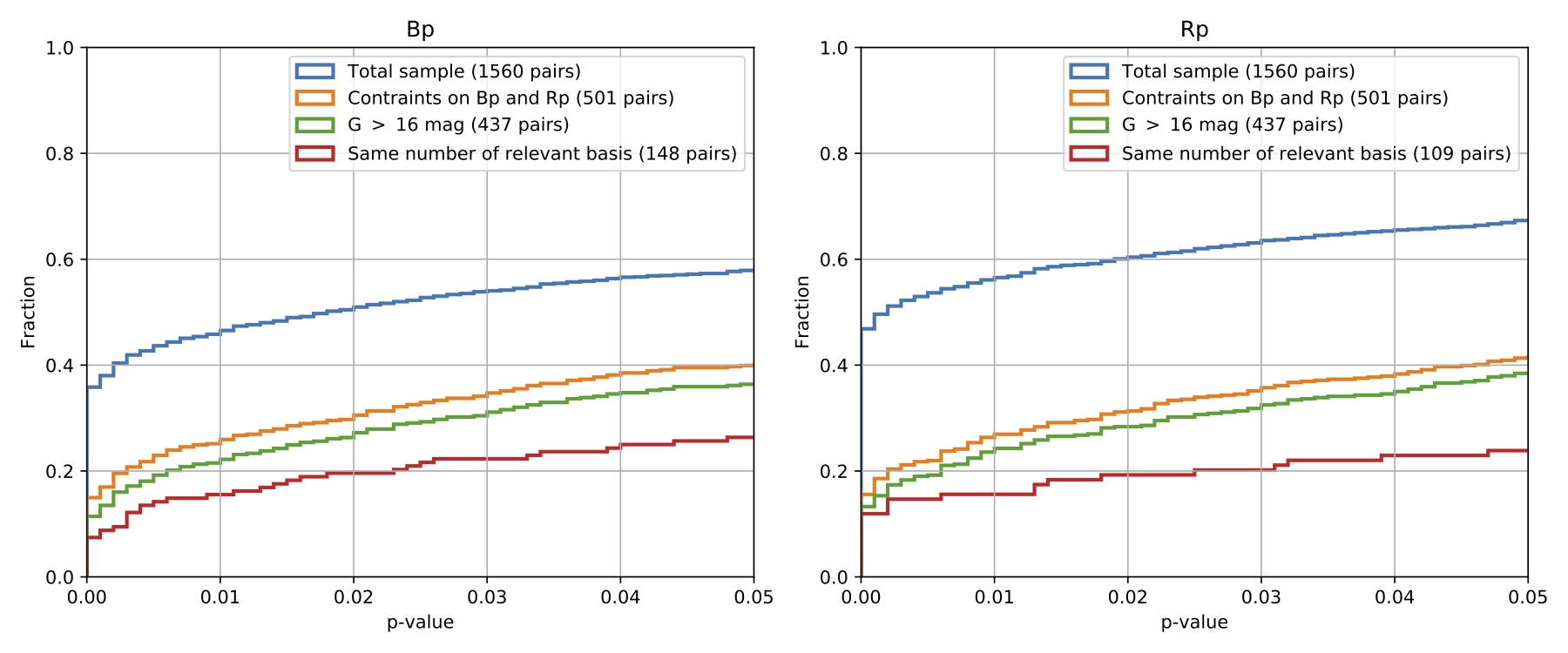Figure 14.90: The panels show the p-value distributions obtained for the two bands. The pairs with a p-value below 0.01 are failing the test.

The $\chi^{2}$ values are then used to calculate the p-values, separately for the BP and RP channels. The cumulative histograms of the p-value distributions of the 1560 stellar pairs are shown in Figure 14.90 (blue lines). The 49$\%$ and 56$\%$ of the pairs have p-value below 0.01 (our failing criterion) in BP and RP, respectively. This indicates that the uncertainties of the XP coefficients are underestimated.

As a second test, we apply more a stringent criteria in the selection of the pairs to be tested. Specifically, we impose that the $G$, $G_{\rm BP}$ and $G_{\rm RP}$ magnitudes of the two stars must be consistent within their uncertainties. This reduces our sample to 501 pairs. The relative p-value distributions are plotted as orange lines in Figure 14.90: the 25$\%$ and 26$\%$ of these pairs fail our test in BP and RP, respectively.

When we apply the further condition that G_1 and G_2 must be lower than 16 mag, we further reduce the sample to 437 pairs. Their p-value distributions are plotted as green lines in Figure 14.90: the fraction of pairs failing our test is now decreased to 22$\%$ and 24$\%$ in BP and RP, respectively. In other words, 50$\%$ of stars that we have removed because brighter than 16 mag were failing our test, while our expectation was to find that only the 25$\%$ of them were failing the test. This indicates that brighter stars have higher chances of failing our test and having underestimate coefficients’ uncertainties.

Finally, study the p-value distributions obtained from the pairs composed by stars with the same number of bp_n_relevant_bases and rp_n_relevant_bases. By doing so we aim at comparing spectra with similar wiggling level. Applying this criteria for the two bands separately we obtain sample of 148 and 109 pairs for BP and RP, respectively. The cumulative distributions are plotted in Figure 14.90 as red lines. The fraction of stars that do not pass the test decreases to 21$\%$ for BP and 15$\%$ for RP, but it is still significant.

In order to estimate how much the errors are underestimate, we multiplied the covariance matrix for a factor equal to 1.1, 1.2, 1.5, 2., and 3. Then we repeated the experiment on the 437 pairs fainter than $G$ =16 mag. The resulting p-value distributions are shown in Figure 14.90 from which we can conclude that the errors have been underestimated of a factor that is between 1.2 and 1.5.